# Subtraction Problems

#### Number of problems found: 62

• Change per hour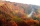On Sunday, the temperature was 63 degrees at 2 pm. The temperature was then 72 degrees at 5 pm. What is the rate of change per hour?
• From a 2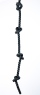From a rope that is 11 m long, two pieces of lengths 13/5 m and 33/10 m are cut off. What is the length of the remaining rope?
• Chin swim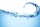Chin swim 9 3/4 miles a week so far this week he was swum 3 1/4 miles. How many more miles does Chin need to swim this week?
• The temperature 16The temperature at 6 am was 21 degrees Fahrenheit by noon, and the temperature dropped 32 degrees Fahrenheit. What was the temperature at noon?
• Product and sumWhat is the product of two fourths  and the sum of three halves and four?About 6/9 of the sixth- grade pupils will be going to the parents' seminar. If 1/6 of the participants are girls, what part of the portion of sixth graders are boys?
• Chocolate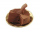State had 906 candies of chocolate. Zoe had 969. how many chocolate did they have in all use addition subtraction and multiplying?
• Benhur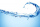Benhur boiled 1 1/4 liters of water in a kettle. After 10 1/2 minutes he measured the water again. It had 3/4 liters left in the kettle. What is the amount of water that evaporates every minutes?
• Jose studiedJose studied for 4 and 1/2 hours on Saturday and another 6 and 1/4 hours on Sunday. How many subjects did he study if he has alloted 1 and 1/2 hours per subject on Saturday and 1 and 1/4 hours per subject on Sunday?
• SubtractSubtract two mixed numbers: 3 1/2 -1 3/4
• SundarSundar has 50 chocolates. He gave 2/5 of these chocolates to Ram and he ate 1/5 of them. How many chocolates are left with Sundar?
• The cashierMarissa bought a dress worth 1966.99. If she gave 2000.00 to the cashier. How much is her change?
• The temp rangeWhat is the range in temperatures if the high temperatures is 67 degrees Celsius and the low temperature is -25 degrees Celsius?
• The temperature 14The temperature is 12°C lower than 4 hours ago. a. What is the average temperature change per hour? b. Write an integer equation for the situation.
• Translate 2Translate the given phrases to mathematical phrases. Thrice the sum of three fifths and two thirds less one half is what number?
• Sum of 20Sum of the two numbers is 15 1/6. If one of the numbers is 2 2/3, find the other number.
• Equation with mixed 2A number, X, is subtracted from 8 1/4. The result is 12 3/5. What is the value of X?
• Hotel 4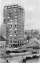A 360 room hotel has 1/3 of its rooms occupied at present. How many rooms are empty?
• The level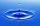The level of temperature of the liquid in a thermometer is 24.32°C lower than the boiling point of water. What is the thermometer reading? Show your answer by drawing and shading the thermometer.
• Bucket of clay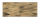Tina and Bill share a 12-ounce bucket of clay. By the end of the week, Tina has used 1/6 of the bucket, and Bill has used 2/3 of the bucket of clay. How many ounces are left in the bucket?

Do you have an exciting math question or word problem that you can't solve? Ask a question or post a math problem, and we can try to solve it.

We will send a solution to your e-mail address. Solved examples are also published here. Please enter the e-mail correctly and check whether you don't have a full mailbox.